# Linear Graph – Definition with Examples

## What is a linear graph?

It is a graphical representation that discusses the relationship between two or more quantities or things. The word ‘linear’ means straight. Linear graphs are straight line graphs to represent the relationship between two quantities.

This graph helps in depicting a result in single straight lines. There is no use of curves, dots, bars, etc., and a straight line is denoted by the term linear.

Let’s represent the given example in the form of a data table.

## Representation

We can represent the distance covered by the object in the y-axis and the time in the x-axis. If we plot the data and join the coordinates, we obtain a straight line.

This type of graph is called a linear graph.

## Different Parts of a Linear Graph

Y-axis: The Y-axis is in the vertical direction, and this is the axis that normally displays a measurement. All the measurements should be of equal distance in this segment if you want to count items such as boxes and ice creams.

X-axis: The X-axis lies on the horizontal line, on which it will represent common names, places, and dates, among other things that need to be analysed. For example, lunchtime, playtime, etc.

The title: When a reader looks at your graph, the first thing they notice is the title. The title should be crisp and to the point, and it should not mention anything useless. For example, the title “lunchboxes in school.” It can also include dates and other information in the graph.

## Linear Graph Equation

This graph forms a straight line and is denoted by the equation:

y = mx + c

where m is the gradient of the graph and c is the y-intercept of the graph.

The linear equation can also be written as,

ax + by + c = 0

where a, b and c are constants.

## Properties of Linear Graph Equations

• A linear equation has two variables with many solutions.
• It can extend to an infinite number of points on the line.
• You can plot as many points as you like to draw this graph, but the minimum number of points needed to plot the correct graph is 2.

## How to Plot a Linear Equation on a Graph

Step 1: Calculate the value of y with respect to x by using the given linear equation.

Step 2: Present these values in a tabular form.

Step 3: Plot the points given in the table in a graph.

Step 4: Join the points and draw a straight line.

## How Is a Linear Graph Different from a Line Graph?

A. Line graph

B. Linear graph

If we compare the two images, we can see that they are quite different. Graph A is a line graph, while graph B is a linear graph. Both these graphs are made up of line segments, but there is a difference between them.

The figure shows the difference after putting the results into a combination of line segments. In this graph all of the points are collinear, so they lie on a line, and this may or may not be the case in a line graph.

## Solved Examples

Example 1: What is a graph with a single line called?

Solution: A graph with a single line is called a simple linear graph.

Example 2: All the points in a linear graph are_____________.

Solution: All the points in a linear graph are collinear.

Example 3: Substitute -2 for x and find the result for y in the equation y = 3x + 1.

Solution: y = 3x + 1

y = 3 (-2) + 1

y = -6 + 1

y =  -5

## Practice Problems

1

### Which of the following are NOT linear graph examples?

A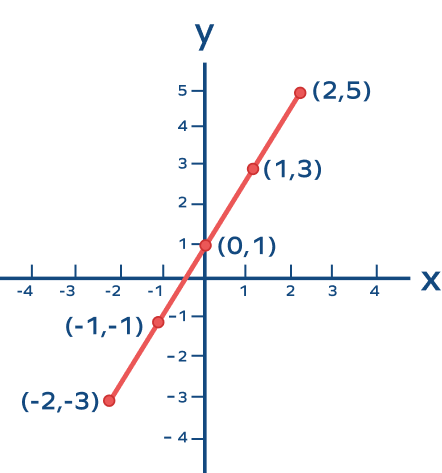B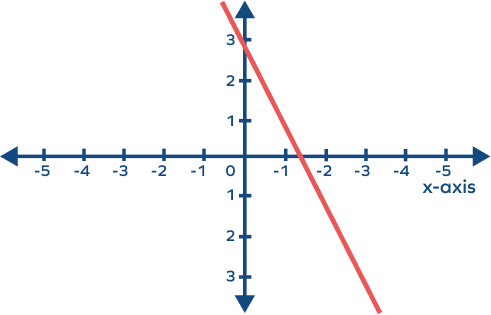C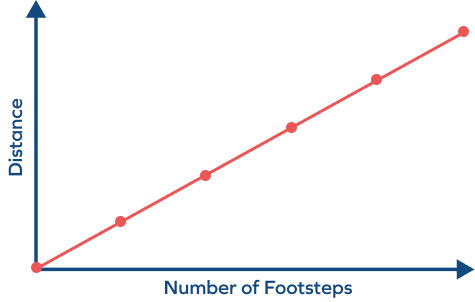D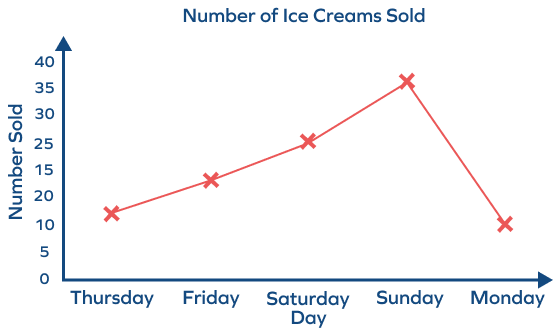CorrectIncorrect
The coordinate points are not collinear.2

### Which of the following is NOT a linear equation?

5x = 6 + 3y
y = 2x + 1
$3x^2+ 2x + 1 = 0$
y = 3x + 2
CorrectIncorrect
Correct answer is: $3x^2+ 2x + 1 = 0$
3

### Which of the following is the graph for the equation y = x?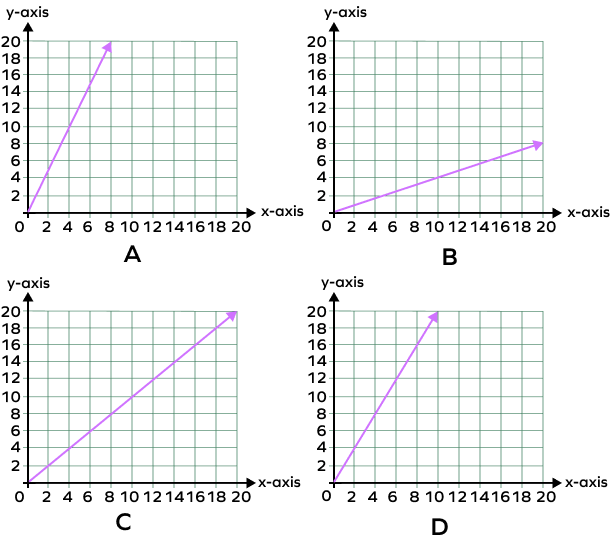A
B
C
D
CorrectIncorrect
According to the given equation x = y,
if x = 2, then y = 2. Graph c) follows the given relation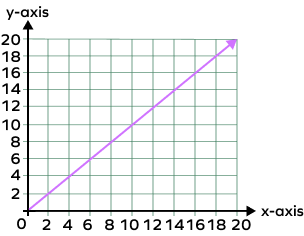## Conclusion

In this lesson, we learned about the use of linear graphs. Graphs can help us represent different activities using lines, and a linear graph is very different from a line graph. Graphs can be very useful for students to learn and understand many different things without getting confused.